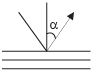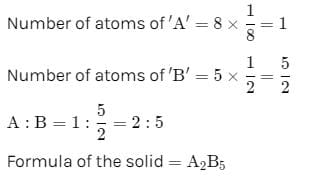Test: Solid State - 2

# Test: Solid State - 2

Test Description

## 30 Questions MCQ Test Physical Chemistry | Test: Solid State - 2

Test: Solid State - 2 for Chemistry 2023 is part of Physical Chemistry preparation. The Test: Solid State - 2 questions and answers have been prepared according to the Chemistry exam syllabus.The Test: Solid State - 2 MCQs are made for Chemistry 2023 Exam. Find important definitions, questions, notes, meanings, examples, exercises, MCQs and online tests for Test: Solid State - 2 below.
Solutions of Test: Solid State - 2 questions in English are available as part of our Physical Chemistry for Chemistry & Test: Solid State - 2 solutions in Hindi for Physical Chemistry course. Download more important topics, notes, lectures and mock test series for Chemistry Exam by signing up for free. Attempt Test: Solid State - 2 | 30 questions in 90 minutes | Mock test for Chemistry preparation | Free important questions MCQ to study Physical Chemistry for Chemistry Exam | Download free PDF with solutions
 1 Crore+ students have signed up on EduRev. Have you?
Test: Solid State - 2 - Question 1

### A solid has a structure in which W-atoms are located at the corners of a cubic lattice, O-atoms at the centre of edges and Na atom at the centre of the cube. The formula for the compound is

Test: Solid State - 2 - Question 2

### The density of CaF2 (fluorite structure) is 3.18 g/cm3. The length of the side of the unit cell is:

Detailed Solution for Test: Solid State - 2 - Question 2

d = zM/NA × V
For flourite structure, z = 4 and M = 78
3.18 = (4 × 78)/6.023 × 1023 × a3
a3 = 312/(6.023×1023 × 3.18)
a3 = 16.28 × 10-23
a = 546 pm

Test: Solid State - 2 - Question 3

### If the anions (A) form hexagonal closest packing and cations (C) occupy only 2/3 octahedral voids in it, then the general formula of the compound is

Test: Solid State - 2 - Question 4

A solid is formed and it has three types of atoms X, Y, Z. X forms a FCC lattice with Y atoms occupying all the tetrahedral voids and Z atoms occupying half the octahedral voids. The formula of the solid is:

Test: Solid State - 2 - Question 5

Which of the following will show Schottky defect?

Detailed Solution for Test: Solid State - 2 - Question 5

The Schottky defects are more common in ionic compounds with high coordination numbers and where the positive and negative ions are of similar size.

Examples include NaCl, CsCl, KCl, KBr and AgBr. Both Schottky and Frenkel defects are observed in AgBr.

Frenkel defects are observed in ZnS, AgCl, AgBr and AgI.

Test: Solid State - 2 - Question 6

In FCC unit cell, what fraction of edge is not covered by atoms:

Test: Solid State - 2 - Question 7

If edge length of the unit cell is 356 pm, then radius of carbon atom in diamond:

Test: Solid State - 2 - Question 8

In a cubic unit cell, the angle between normal to the planes (111) and (121) is:

Test: Solid State - 2 - Question 9

How many unit cells are present in a cube-shaped ideal crystal of NaCl of mass 1.0 g?

Test: Solid State - 2 - Question 10

Co-ordination no. of ‘c’ in Be2C3 whose structure is co-related with CaF2 is:

Test: Solid State - 2 - Question 11

The value of d111 in a cubic crystal is 325.6 pm. The value of d333 is (d = interplaner spacing):

Test: Solid State - 2 - Question 12

X-rays of wKa (wavelength 154 pm) are diffracted by a set of atomic planes in a crystals in the following manner. The separation of the la yers in the crystals in 404 Pm. Find the angle a along which the first order reflection will occur:Test: Solid State - 2 - Question 13

In Rocksalt structure:

Test: Solid State - 2 - Question 14

In a fcc lattice, atom A occupies the corner position and atom B occupies the face centre position. If one atom of B is missing from one of the face centred points, the formula of the compound is:

Detailed Solution for Test: Solid State - 2 - Question 14Test: Solid State - 2 - Question 15

The angle between the two planes represented by the Miller indices (110) and (111) in a simple cubic:

Detailed Solution for Test: Solid State - 2 - Question 15

cosθ = (h1h2 + k1k2 + l1l2)/(h12 + k12 + l12)1/2(h22+ k22 + l22)1/2

=> (1+1+0)/[(12 + 12 + 02)1/2(12 + 12 + 02)½]

=> 2/(6)1/2

=> cos-1 2/(6)1/2

=> 30o approx.

Test: Solid State - 2 - Question 16

The coordinate number for an atom in a primitive cubic unit cell is

Detailed Solution for Test: Solid State - 2 - Question 16

The coordination number for an atom in a primitive cubic unit cell is 6. Each sphere present at a corner of the unit cell is surrounded by 6 neighboring spheres present at 6 other corners.

Test: Solid State - 2 - Question 17

The three dimensional graph of lattice points which sets the pattern for the whole lattice is called

Test: Solid State - 2 - Question 18

When Frenkel defects are created in an otherwise perfect ionic crystal, the density of the ionic crystal:

Test: Solid State - 2 - Question 19

The compound formed by elements X and Y crystallizes in cubic structure in which X atoms are at the corners of the cube and Y atoms are at the face centre . The formula of the compound is

Test: Solid State - 2 - Question 20

The angle at which the first order Bragg reflection is observed from (110) plane in a simple cubic unit cell of side 3.238 Å when chromium Ka radiation of wavelength 2.29 Å is used, is:

Test: Solid State - 2 - Question 21

Number of rotational symmetry axes for triclinic crystal system is:

Test: Solid State - 2 - Question 22

The lattice parameter of an element stabilized in a fcc structure is 4.04 Å. The atomic radius of the element is:

Test: Solid State - 2 - Question 23

A metal crystallizes in fcc structure with a unit cell side of 500 pm. If the density of the crystal is 1.33 g/cc, the molar mass of the metal is closed to:

Test: Solid State - 2 - Question 24

Percentage of free space in a body centred cubic unit cell is

Test: Solid State - 2 - Question 25

An element exists in two crystallographic modifications with FCC and BCC structures. The ratio of the densities of the FCC and BCC modifications in terms of the volumes of their unit cells (VFCC and VBCC) is:

Test: Solid State - 2 - Question 26

Metallic gold crystallizes in fcc structure with unit cell dimension of 4.00 Å The atomic radius.

Test: Solid State - 2 - Question 27

Both NaCl and KCl crystallize with Fcc structure. However, the X-ray powder diffraction pattern corresponds to the fcc structure whereas, that, of KCl corresponds to sample cubic structure. This is because:

Test: Solid State - 2 - Question 28

In a simple cubic, body-centred cubic and face-centred cubic structure, the ratio of the number of atoms present is respectively

Detailed Solution for Test: Solid State - 2 - Question 28

Face centered cube consists of eight atoms at the corners of the cube and six atoms at the center of each face. Hence, the ratio of number of atoms present in a simple cubic, body centered cubic and face centered cubic structures is 1 : 2 : 4.

Test: Solid State - 2 - Question 29

The interplanar spacing of (110) planes in a cubic unit cell with lattice parameter a = 4.242 Å is:

Test: Solid State - 2 - Question 30

The edge length of a face centred cubic cell of an ionic substance is 508 pm. If the radius of the cation is 110 pm, the radius of the anion is

## Physical Chemistry

85 videos|131 docs|67 tests
 Use Code STAYHOME200 and get INR 200 additional OFF Use Coupon Code
Information about Test: Solid State - 2 Page
In this test you can find the Exam questions for Test: Solid State - 2 solved & explained in the simplest way possible. Besides giving Questions and answers for Test: Solid State - 2, EduRev gives you an ample number of Online tests for practice

## Physical Chemistry

85 videos|131 docs|67 tests